Function Repository Resource:

# WolframModelRuleForm

Represent a Wolfram model in x, y, … letter form

Contributed by: Stephen Wolfram
 ResourceFunction["WolframModelRuleForm"][rule] converts a Wolfram model specification to x, y, … letter form.

## Details and Options

A heuristic is used to label the nodes canonically.
If the rule contains numbers greater than 50, the representation is kept in a numeric form.

## Examples

### Basic Examples (1)

Some Wolfram models represented in rule form:

 In:=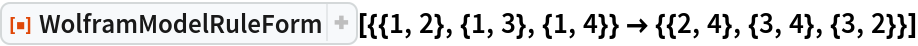Out=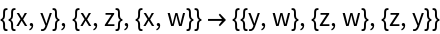In:=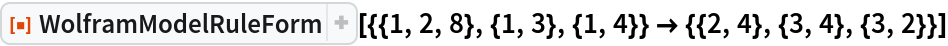Out=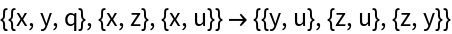### Scope (2)

Greek letters are used when the numbers in the rule are more than 26:

 In:=Out=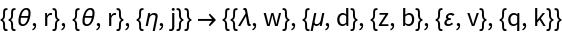When the numbers in the rule exceed 50, the rule is kept in numeric form:

 In:=Out=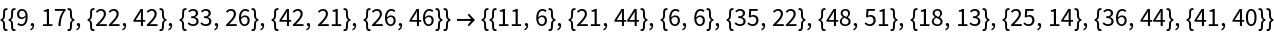## Version History

• 2.0.0 – 24 March 2020
• 1.0.0 – 16 March 2020